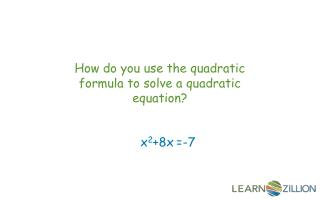DownloadDownload PresentationHow do you use the quadratic formula to solve a quadratic equation?

# How do you use the quadratic formula to solve a quadratic equation?

Download Presentation## How do you use the quadratic formula to solve a quadratic equation?

- - - - - - - - - - - - - - - - - - - - - - - - - - - E N D - - - - - - - - - - - - - - - - - - - - - - - - - - -
##### Presentation Transcript

1. How do you use the quadratic formula to solve a quadratic equation? x2+8x=-7

2. In this lesson you will learn to solve a quadratic equation by the quadratic formula.

3. We can derive the quadratic formula by completing the square

4. The sign of a number is really important! -(-3) = 3 (-3)2 = 9

5. Correctly identifying a, b and c ax2+bx+c=0 a=1 b=3 c=5 a=1 b=3 c=-1 a=1 b=3 c=5

6. Solve the equation using the quadratic formula. 2x2-3x= -1/2 ax2+bx+c=0 Where are the a, b and c? 2x2 - 3x = -1/2 c= a=2 b=-3

7. Substitution

8. In this lesson you have learned how to solve a quadratic equation by using the quadratic formula.

9. Solve this quadratic by factoring, completing the square and the quadratic formula. How do the solutions compare? x2+13x+33=0

10. Describe a situation where the quadratic formula would yield only one real solution to a quadratic equation.

11. Write a quadratic equation where the standard form of the quadratic has c=0. Solve the following by the quadratic formula. 3x2+8x-19=0